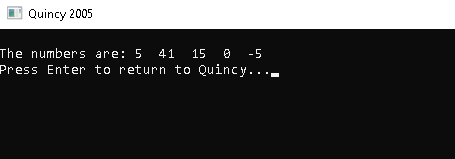# Array

#### Array definition and declaration

Arrays are a sort of data structure that may store a definite number of items of the same type in a logical order. Although an array is used to store data, it is often more convenient to think of it as a collection of similar-type variables.

You create a single array variable named numbers and use numbers, numbers,..., numbers to represent individual variables instead of individual variables like number0, number1,..., and number99. In an array, an index is used to retrieve a specific element.

#### Declaring Arrays

In C, a programmer declares an array by specifying the type of components and the number of elements required by the array as follows:

#### Syntax

dataType arrayname[arraysize];

A single-dimensional array is what this is termed. arraySize must be a positive integer constant, and type can be any acceptable C data type. Use the expression double balance to declare a 10-element array of type double named balance.

#### Declaring Arrays

• Array variables are defined similarly to data type variables, with the difference that each array dimension's variable name is preceded by one pair of square [ ] brackets.
• The size of rows, columns, and other elements of uninitialized arrays must be specified within square brackets.
• When defining arrays in C, the dimensions must be positive integral constants or constant expressions.
• Variables can be used as long as they have a positive value when the array is declared, but dimensions must still be positive integers in C99. (When the array is defined, space is only allocated once.) The array does not change in size if the variable used to define it changes later.

int x;

#### Example :

#include <iostream> using namespace std; int main() { int x = {5, 41, 15, 0, -5}; cout << "\nThe numbers are: "; // Printing array elements using intex for (int i = 0; i < 5; ++i) { cout << x[i] << " "; } return 0; }

#### OUTPUT: